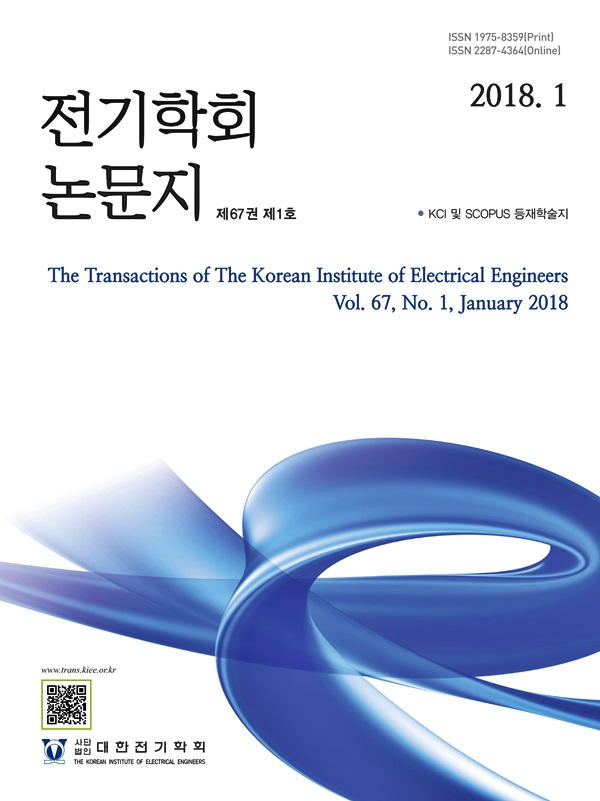Mobile QR Code#### The Transactions of the Korean Institute of Electrical Engineers

##### ISO Journal TitleTrans. Korean. Inst. Elect. Eng.
• SCOPUS
• KCI Accredited Journal
Title Development of Partial Discharge Analysis Model by Void in Solid Insulation Using Nonlinear Characteristics of Black-box Arc Model 주형준(Hyung-Jun Ju) ; 이종건(Jong-Geon Lee) ; 한기선(Ki-Sun Han) ; 박규훈(Kyu-Hoon Park) ; 이방욱(Bang-Wook Lee) https://doi.org/10.5370/KIEE.2020.69.11.1707 pp.1707-1716 1975-8359 Partial Discharge Simulation; Void; Capacitive Equivalent model; Black-Box Arc Model; Nonlinear Characteristics Partial discharge measurement and analysis of solid insulators should be performed indispensably for predicting the insulation life and diagnosis of electric power facilities. Prior to the actual partial discharge measurement, the partial discharge can be analyzed through a simulation study based on the design of an accurate equivalent model of the insulating materials. The high reliability of partial discharge simulation can reduce cost, manpower, and time required when performing actual partial discharge measurement tests, and can be effectively applied to complex partial discharge characteristics analysis of insulating materials. In this paper, a partial discharge simulation was performed by designing an equivalent model of a partial discharge test cell injected with a small circular void in the inner center. To evaluate the reliability of the simulation results, the actual partial discharge of the test cell was measured according to the test procedure of IEC-60270. A capacitive equivalent model that can simulate the partial discharge characteristics of the test cell was designed and a simulation study of partial discharge inside the insulator was conducted. The partial discharge measurement system is designed using a black-box arc model that can describe the nonlinear characteristics of the arc. The parameters of the simulation model were selected by establishing a simulation scenario assuming the condition of adding electrical deterioration to the voids of the same size. In addition, to verify the simulation results, PRPD (Phase Resolved Partial Discharge) patterns generated in voids was obtained using a partial discharge measuring device. The parameters related to the partial discharge characteristics such as the maximum partial discharge amplitude, number and distribution were investigated. As a result, the consistency of the partial discharge simulation results was verified through a comparative study of simulation and test results, and a reliable partial discharge modeling technique was established.